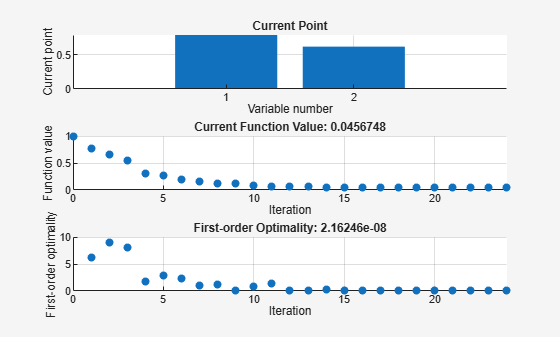Main Content

## Plot Functions

### Plot an Optimization During Execution

You can plot various measures of progress during the execution of a solver. Set the `PlotFcn` name-value pair in `optimoptions`, and specify one or more plotting functions for the solver to call at each iteration. Pass a function handle or cell array of function handles.

There are a variety of predefined plot functions available. See the `PlotFcn` option description in the solver function reference page.

You can also use a custom-written plot function. Write a function file using the same structure as an output function. For more information on this structure, see Output Function and Plot Function Syntax.

### Use a Plot Function

This example shows how to use plot functions to view the progress of the `fmincon` `'interior-point'` algorithm. The problem is taken from Solve a Constrained Nonlinear Problem, Solver-Based.

Write the nonlinear objective and constraint functions, including their gradients. The objective function is Rosenbrock's function.

`type rosenbrockwithgrad`
```function [f,g] = rosenbrockwithgrad(x) % Calculate objective f f = 100*(x(2) - x(1)^2)^2 + (1-x(1))^2; if nargout > 1 % gradient required g = [-400*(x(2)-x(1)^2)*x(1)-2*(1-x(1)); 200*(x(2)-x(1)^2)]; end ```

Save this file as `rosenbrockwithgrad.m`.

The constraint function is that the solution satisfies `norm(x)^2 <= 1`.

`type unitdisk2`
```function [c,ceq,gc,gceq] = unitdisk2(x) c = x(1)^2 + x(2)^2 - 1; ceq = [ ]; if nargout > 2 gc = [2*x(1);2*x(2)]; gceq = []; end ```

Save this file as `unitdisk2.m`.

Create an options structure that includes calling three plot functions:

```options = optimoptions(@fmincon,'Algorithm','interior-point',... 'SpecifyObjectiveGradient',true,'SpecifyConstraintGradient',true,... 'PlotFcn',{@optimplotx,@optimplotfval,@optimplotfirstorderopt});```

Create the initial point `x0 = [0,0]`, and set the remaining inputs to empty (`[]`).

```x0 = [0,0]; A = []; b = []; Aeq = []; beq = []; lb = []; ub = [];```

Call `fmincon`, including the options.

```fun = @rosenbrockwithgrad; nonlcon = @unitdisk2; x = fmincon(fun,x0,A,b,Aeq,beq,lb,ub,nonlcon,options)``````Local minimum found that satisfies the constraints. Optimization completed because the objective function is non-decreasing in feasible directions, to within the value of the optimality tolerance, and constraints are satisfied to within the value of the constraint tolerance. ```
```x = 1×2 0.7864 0.6177 ```

Download now